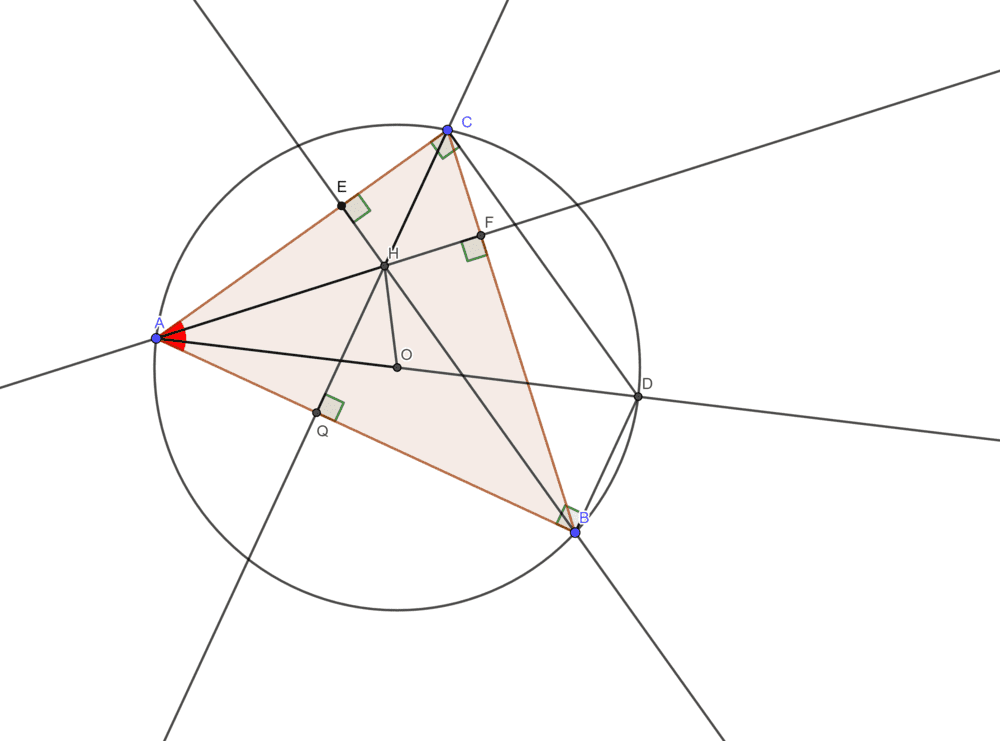# Find this angle given the triangle's Orthocenter

• kaloyan

#### kaloyan

Homework Statement
An acute ##\triangle ABC##, inscribed in a circle ##k## with radii ##R##, is given. Point ##H## is the orthocenter of ##\triangle ABC## and ##AH=R##. Find ##\angle BAC##. (Answer: ##60^\circ##)
Relevant Equations
-##AD## is diameter, thus ##\angle ACD = \angle ABD = 90^\circ##. Also ##HBDC## is a parallelogram because ##HC||BD, HB||CD##. It seems useless and I don't know how to continue. Thank you in advance!

What drawing program did you use to make that figure?

What drawing program did you use to make that figure?
I've used GeoGebra.

This actually doesn't have one answer. just put A=70 for example, and it won't go wrong, or 60, and etc.

This actually doesn't have one answer. just put A=70 for example, and it won't go wrong, or 60, and etc.
I don't get what you are trying to say. If you try to draw the same picture with an angle of ##70^\circ## you don't get the lengths ##AH = AO##.

I don't get what you are trying to say. If you try to draw the same picture with an angle of ##70^\circ## you don't get the lengths ##AH = AO##.
Oh, yes, you are right. For reasons, I thought any angle would be correct

Problem Statement: An acute ##\triangle ABC##, inscribed in a circle ##k## with radii ##R##, is given. Point ##H## is the orthocenter of ##\triangle ABC## and ##AH=R##. Find ##\angle BAC##. (Answer: ##60^\circ##)
Relevant Equations: -

View attachment 243002
##AD## is diameter, thus ##\angle ACD = \angle ABD = 90^\circ##. Also ##HBDC## is a parallelogram because ##HC||BD, HB||CD##. It seems useless and I don't know how to continue. Thank you in advance!
So, you can continue by showing first portion A is equal to the third portion A, Then try to use trigonometry since AH=AO=R and AD=2R, and then you will get the answer.

So, you can continue by showing first portion A is equal to the third portion A, Then try to use trigonometry since AH=AO=R and AD=2R, and then you will get the answer.
I'm 8th grade - I do not know Trigonometry.

I'm 8th grade - I do not know Trigonometry.
What about similarity? It can be solved by it.(I don't know how is your country's education system)
And another clue to solve the problem: After you showed that the first portion A is equal to the third portion A, then by this and by knowing that AH=AO=R and AD=2R , try to prove 2AE=AB (by similarity)